# Object Detection Using Faster R-CNN Deep Learning

This example shows how to train a Faster R-CNN (regions with convolutional neural networks) object detector.

Deep learning is a powerful machine learning technique that you can use to train robust object detectors. Several deep learning techniques for object detection exist, including Faster R-CNN and you only look once (YOLO) v2. This example trains a Faster R-CNN vehicle detector using the `trainFasterRCNNObjectDetector` function. For more information, see Object Detection using Deep Learning.

Download a pretrained detector to avoid having to wait for training to complete. If you want to train the detector, set the `doTrainingAndEval` variable to true.

```doTrainingAndEval = false; if ~doTrainingAndEval && ~exist('fasterRCNNResNet50EndToEndVehicleExample.mat','file') disp('Downloading pretrained detector (118 MB)...'); pretrainedURL = 'https://www.mathworks.com/supportfiles/vision/data/fasterRCNNResNet50EndToEndVehicleExample.mat'; websave('fasterRCNNResNet50EndToEndVehicleExample.mat',pretrainedURL); end```

This example uses a small labeled dataset that contains 295 images. Each image contains one or two labeled instances of a vehicle. A small dataset is useful for exploring the Faster R-CNN training procedure, but in practice, more labeled images are needed to train a robust detector. Unzip the vehicle images and load the vehicle ground truth data.

```unzip vehicleDatasetImages.zip data = load('vehicleDatasetGroundTruth.mat'); vehicleDataset = data.vehicleDataset;```

The vehicle data is stored in a two-column table, where the first column contains the image file paths and the second column contains the vehicle bounding boxes.

Split the dataset into training, validation, and test sets. Select 60% of the data for training, 10% for validation, and the rest for testing the trained detector.

```rng(0) shuffledIndices = randperm(height(vehicleDataset)); idx = floor(0.6 * height(vehicleDataset)); trainingIdx = 1:idx; trainingDataTbl = vehicleDataset(shuffledIndices(trainingIdx),:); validationIdx = idx+1 : idx + 1 + floor(0.1 * length(shuffledIndices) ); validationDataTbl = vehicleDataset(shuffledIndices(validationIdx),:); testIdx = validationIdx(end)+1 : length(shuffledIndices); testDataTbl = vehicleDataset(shuffledIndices(testIdx),:);```

Use `imageDatastore` and `boxLabelDatastore` to create datastores for loading the image and label data during training and evaluation.

```imdsTrain = imageDatastore(trainingDataTbl{:,'imageFilename'}); bldsTrain = boxLabelDatastore(trainingDataTbl(:,'vehicle')); imdsValidation = imageDatastore(validationDataTbl{:,'imageFilename'}); bldsValidation = boxLabelDatastore(validationDataTbl(:,'vehicle')); imdsTest = imageDatastore(testDataTbl{:,'imageFilename'}); bldsTest = boxLabelDatastore(testDataTbl(:,'vehicle'));```

Combine image and box label datastores.

```trainingData = combine(imdsTrain,bldsTrain); validationData = combine(imdsValidation,bldsValidation); testData = combine(imdsTest,bldsTest);```

Display one of the training images and box labels.

```data = read(trainingData); I = data{1}; bbox = data{2}; annotatedImage = insertShape(I,'Rectangle',bbox); annotatedImage = imresize(annotatedImage,2); figure imshow(annotatedImage)```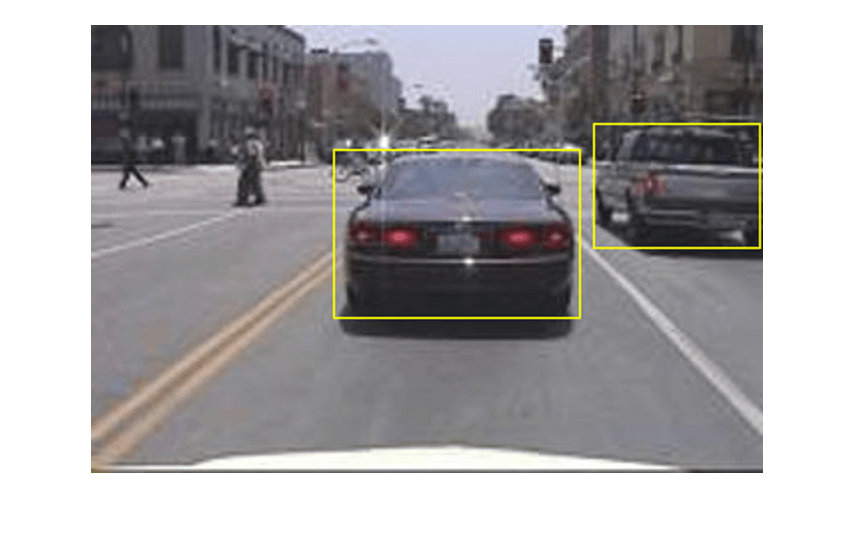### Create Faster R-CNN Detection Network

A Faster R-CNN object detection network is composed of a feature extraction network followed by two subnetworks. The feature extraction network is typically a pretrained CNN, such as ResNet-50 or Inception v3. The first subnetwork following the feature extraction network is a region proposal network (RPN) trained to generate object proposals - areas in the image where objects are likely to exist. The second subnetwork is trained to predict the actual class of each object proposal.

The feature extraction network is typically a pretrained CNN (for details, see Pretrained Deep Neural Networks (Deep Learning Toolbox)). This example uses ResNet-50 for feature extraction. You can also use other pretrained networks such as MobileNet v2 or ResNet-18, depending on your application requirements.

Use `fasterRCNNLayers` to create a Faster R-CNN network automatically given a pretrained feature extraction network. `fasterRCNNLayers` requires you to specify several inputs that parameterize a Faster R-CNN network:

• Network input size

• Anchor boxes

• Feature extraction network

First, specify the network input size. When choosing the network input size, consider the minimum size required to run the network itself, the size of the training images, and the computational cost incurred by processing data at the selected size. When feasible, choose a network input size that is close to the size of the training image and larger than the input size required for the network. To reduce the computational cost of running the example, specify a network input size of [224 224 3], which is the minimum size required to run the network.

`inputSize = [224 224 3];`

Note that the training images used in this example are bigger than 224-by-224 and vary in size, so you must resize the images in a preprocessing step prior to training.

Next, use `estimateAnchorBoxes` to estimate anchor boxes based on the size of objects in the training data. To account for the resizing of the images prior to training, resize the training data for estimating anchor boxes. Use `transform` to preprocess the training data, then define the number of anchor boxes and estimate the anchor boxes.

```preprocessedTrainingData = transform(trainingData, @(data)preprocessData(data,inputSize)); numAnchors = 3; anchorBoxes = estimateAnchorBoxes(preprocessedTrainingData,numAnchors)```
```anchorBoxes = 3×2 136 119 55 48 157 128 ```

For more information on choosing anchor boxes, seeEstimate Anchor Boxes From Training Data (Computer Vision Toolbox™) and Anchor Boxes for Object Detection.

Now, use `resnet50` to load a pretrained ResNet-50 model.

`featureExtractionNetwork = resnet50;`

Select `'activation_40_relu'` as the feature extraction layer. This feature extraction layer outputs feature maps that are downsampled by a factor of 16. This amount of downsampling is a good trade-off between spatial resolution and the strength of the extracted features, as features extracted further down the network encode stronger image features at the cost of spatial resolution. Choosing the optimal feature extraction layer requires empirical analysis. You can use `analyzeNetwork` to find the names of other potential feature extraction layers within a network.

`featureLayer = 'activation_40_relu';`

Define the number of classes to detect.

`numClasses = width(vehicleDataset)-1;`

Create the Faster R-CNN object detection network.

`lgraph = fasterRCNNLayers(inputSize,numClasses,anchorBoxes,featureExtractionNetwork,featureLayer);`

You can visualize the network using `analyzeNetwork` or Deep Network Designer from Deep Learning Toolbox™.

If more control is required over the Faster R-CNN network architecture, use Deep Network Designer to design the Faster R-CNN detection network manually. For more information, see Getting Started with R-CNN, Fast R-CNN, and Faster R-CNN.

### Data Augmentation

Data augmentation is used to improve network accuracy by randomly transforming the original data during training. By using data augmentation, you can add more variety to the training data without actually having to increase the number of labeled training samples.

Use `transform` to augment the training data by randomly flipping the image and associated box labels horizontally. Note that data augmentation is not applied to test and validation data. Ideally, test and validation data are representative of the original data and are left unmodified for unbiased evaluation.

`augmentedTrainingData = transform(trainingData,@augmentData);`

Read the same image multiple times and display the augmented training data.

```augmentedData = cell(4,1); for k = 1:4 data = read(augmentedTrainingData); augmentedData{k} = insertShape(data{1},'Rectangle',data{2}); reset(augmentedTrainingData); end figure montage(augmentedData,'BorderSize',10)```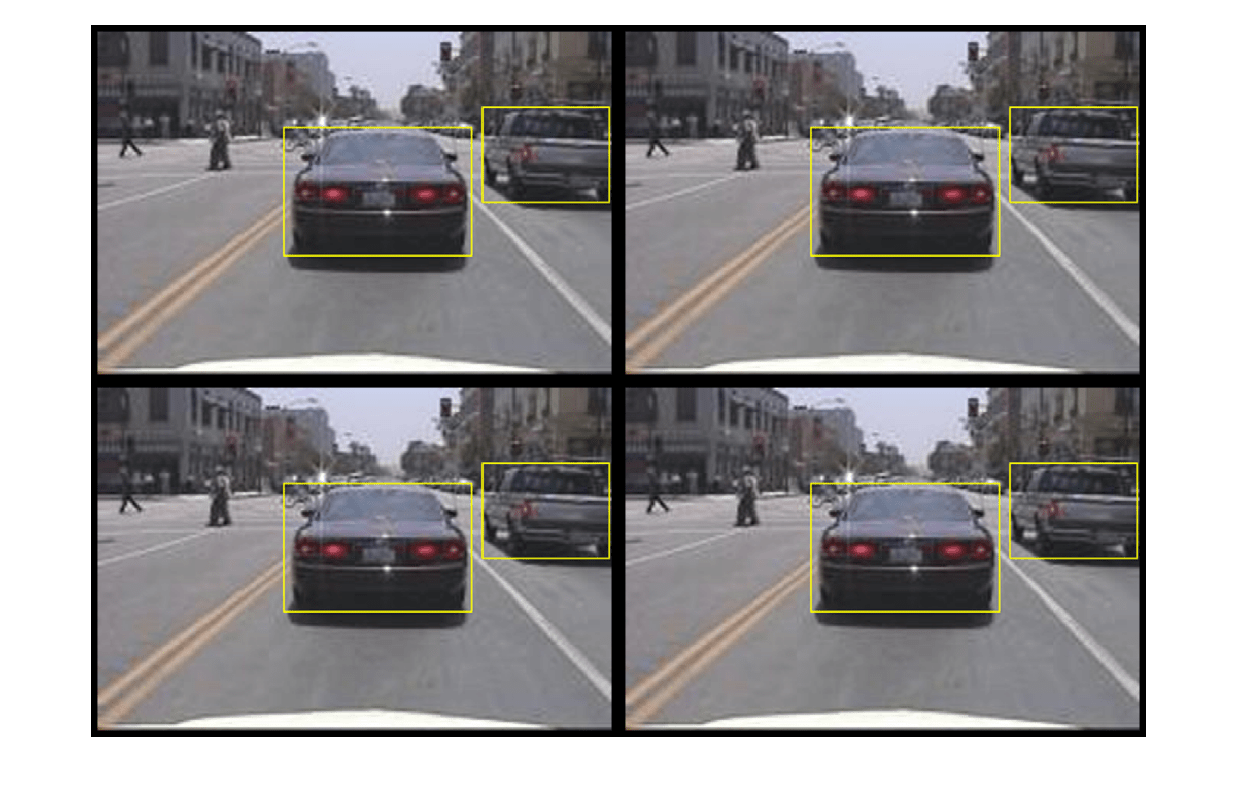### Preprocess Training Data

Preprocess the augmented training data, and the validation data to prepare for training.

```trainingData = transform(augmentedTrainingData,@(data)preprocessData(data,inputSize)); validationData = transform(validationData,@(data)preprocessData(data,inputSize));```

`data = read(trainingData);`

Display the image and box bounding boxes.

```I = data{1}; bbox = data{2}; annotatedImage = insertShape(I,'Rectangle',bbox); annotatedImage = imresize(annotatedImage,2); figure imshow(annotatedImage)```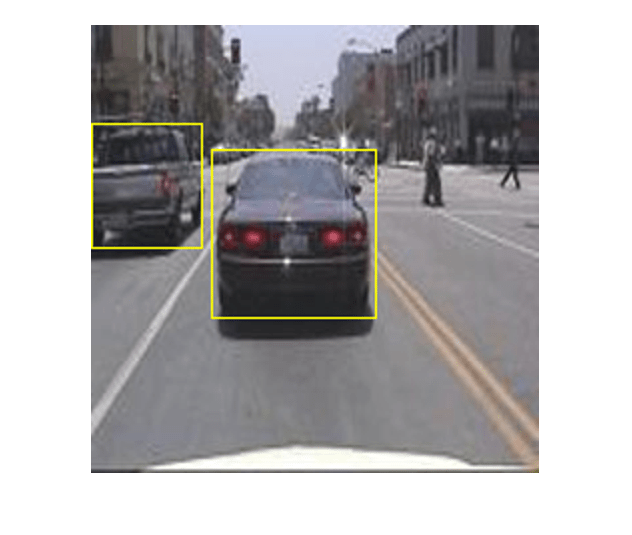### Train Faster R-CNN

Use `trainingOptions` to specify network training options. Set `'ValidationData'` to the preprocessed validation data. Set `'CheckpointPath'` to a temporary location. This enables the saving of partially trained detectors during the training process. If training is interrupted, such as by a power outage or system failure, you can resume training from the saved checkpoint.

```options = trainingOptions('sgdm',... 'MaxEpochs',10,... 'MiniBatchSize',2,... 'InitialLearnRate',1e-3,... 'CheckpointPath',tempdir,... 'ValidationData',validationData);```

Use `trainFasterRCNNObjectDetector` to train Faster R-CNN object detector if `doTrainingAndEval` is true. Otherwise, load the pretrained network.

```if doTrainingAndEval % Train the Faster R-CNN detector. % * Adjust NegativeOverlapRange and PositiveOverlapRange to ensure % that training samples tightly overlap with ground truth. [detector, info] = trainFasterRCNNObjectDetector(trainingData,lgraph,options, ... 'NegativeOverlapRange',[0 0.3], ... 'PositiveOverlapRange',[0.6 1]); else % Load pretrained detector for the example. pretrained = load('fasterRCNNResNet50EndToEndVehicleExample.mat'); detector = pretrained.detector; end```

This example was verified on an Nvidia(TM) Titan X GPU with 12 GB of memory. Training the network took approximately 20 minutes. The training time varies depending on the hardware you use.

As a quick check, run the detector on one test image. Make sure you resize the image to the same size as the training images.

```I = imread(testDataTbl.imageFilename{1}); I = imresize(I,inputSize(1:2)); [bboxes,scores] = detect(detector,I);```

Display the results.

```I = insertObjectAnnotation(I,'rectangle',bboxes,scores); figure imshow(I)```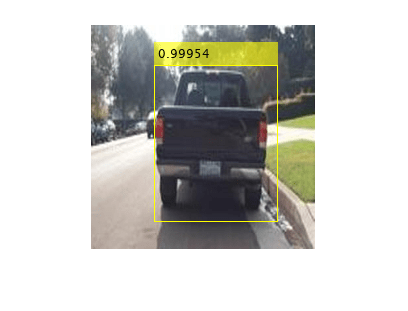### Evaluate Detector Using Test Set

Evaluate the trained object detector on a large set of images to measure the performance. Computer Vision Toolbox™ provides object detector evaluation functions to measure common metrics such as average precision (`evaluateDetectionPrecision`) and log-average miss rates (`evaluateDetectionMissRate`). For this example, use the average precision metric to evaluate performance. The average precision provides a single number that incorporates the ability of the detector to make correct classifications (precision) and the ability of the detector to find all relevant objects (recall).

Apply the same preprocessing transform to the test data as for the training data.

`testData = transform(testData,@(data)preprocessData(data,inputSize));`

Run the detector on all the test images.

```if doTrainingAndEval detectionResults = detect(detector,testData,'MinibatchSize',4); else % Load pretrained detector for the example. pretrained = load('fasterRCNNResNet50EndToEndVehicleExample.mat'); detectionResults = pretrained.detectionResults; end ```

Evaluate the object detector using the average precision metric.

`[ap, recall, precision] = evaluateDetectionPrecision(detectionResults,testData);`

The precision/recall (PR) curve highlights how precise a detector is at varying levels of recall. The ideal precision is 1 at all recall levels. The use of more data can help improve the average precision but might require more training time. Plot the PR curve.

```figure plot(recall,precision) xlabel('Recall') ylabel('Precision') grid on title(sprintf('Average Precision = %.2f', ap))```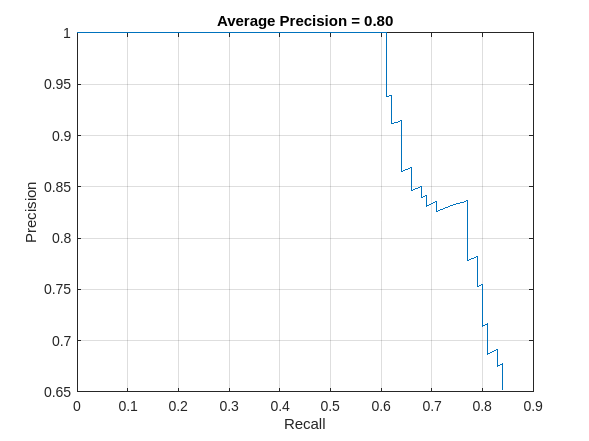### Supporting Functions

```function data = augmentData(data) % Randomly flip images and bounding boxes horizontally. tform = randomAffine2d('XReflection',true); rout = affineOutputView(size(data{1}),tform); data{1} = imwarp(data{1},tform,'OutputView',rout); data{2} = bboxwarp(data{2},tform,rout); end function data = preprocessData(data,targetSize) % Resize image and bounding boxes to targetSize. scale = targetSize(1:2)./size(data{1},[1 2]); data{1} = imresize(data{1},targetSize(1:2)); data{2} = bboxresize(data{2},scale); end```

### References

 Ren, S., K. He, R. Gershick, and J. Sun. "Faster R-CNN: Towards Real-Time Object Detection with Region Proposal Networks." IEEE Transactions of Pattern Analysis and Machine Intelligence. Vol. 39, Issue 6, June 2017, pp. 1137-1149.

 Girshick, R., J. Donahue, T. Darrell, and J. Malik. "Rich Feature Hierarchies for Accurate Object Detection and Semantic Segmentation." Proceedings of the 2014 IEEE Conference on Computer Vision and Pattern Recognition. Columbus, OH, June 2014, pp. 580-587.

 Girshick, R. "Fast R-CNN." Proceedings of the 2015 IEEE International Conference on Computer Vision. Santiago, Chile, Dec. 2015, pp. 1440-1448.

 Zitnick, C. L., and P. Dollar. "Edge Boxes: Locating Object Proposals from Edges." European Conference on Computer Vision. Zurich, Switzerland, Sept. 2014, pp. 391-405.

 Uijlings, J. R. R., K. E. A. van de Sande, T. Gevers, and A. W. M. Smeulders. "Selective Search for Object Recognition." International Journal of Computer Vision. Vol. 104, Number 2, Sept. 2013, pp. 154-171.